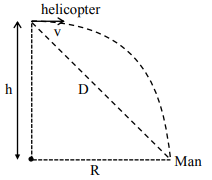# A helicopter is flying horizontally with a speed ' v '`
Question:

A helicopter is flying horizontally with a speed ' $v$ ' at an altitude 'h' has to drop a food packet for a man on the ground. What is the distance of helicopter from the man when the food packet is dropped?

1. $\sqrt{\frac{2 g h v^{2}+1}{h^{2}}}$

2. $\sqrt{2 \mathrm{ghv}^{2}+\mathrm{h}^{2}}$

3. $\sqrt{\frac{2 v^{2} h}{g}+h^{2}}$

4. $\sqrt{\frac{2 g h}{v^{2}}}+h^{2}$

Correct Option: , 3

Solution:$R=\sqrt{\frac{2 h}{g}} \cdot v$

$\mathrm{D}=\sqrt{\mathrm{R}^{2}+\mathrm{h}^{2}}$

$=\sqrt{\left(\sqrt{\frac{2 h}{g}} \cdot v\right)^{2}+h^{2}}$

$D=\sqrt{\frac{2 h v^{2}}{g}+h^{2}}$

Option (3) is correct